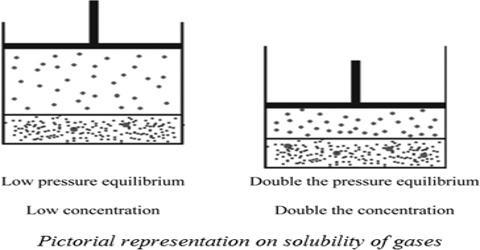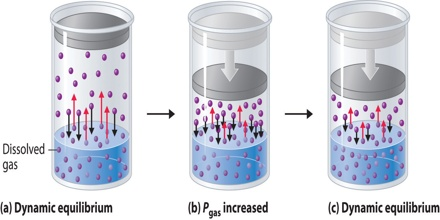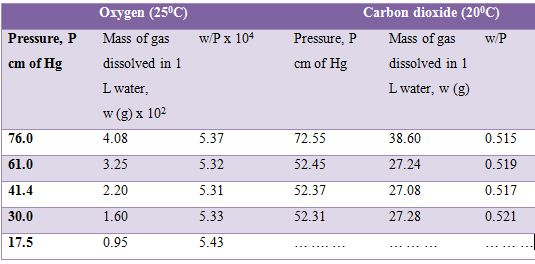# Influence of Pressure on Solubility

Influence of Pressure on Solubility

The solubility of gases in liquids is greatly influenced by pressure and in all cases the solubility increases with increase in pressure. This qualitative statement is expressed quantitatively by Henry’s law which states that “the amount of a gas in g dissolved in a definite volume of solvent is directly proportional to the pressure of the gas as constant temperature”. Mathematically,

w ∞ P

or, w/P = constant

where w is the mass of the gas in g and P is the pressure. The solubility of oxygen and carbon dioxide in water at different pressures are given in Table. It can be seen that the values of w/P are approximately constant for both the gases.Henry’s law may also be stated in two other forms:

(a) Let w g of a gas of volume V mL at a pressure P is dissolved in a given volume of a liquid. If the pressure is increased to 2P, the mass of gas dissolved in the same volume of the liquid will be 2w g according to Henry’s law.

Table: Solubility of oxygen and carbon dioxide in waterIn the case of an ideal gas obeying Boyle’s law the volume of 2w g of gas at pressure P is 2V mL but at pressure 2P it is V mL. Hence it can be stated that-

“The volume of a gas, measured at the pressure of the experiment, dissolved in a given volume of liquid at a constant temperature is independent of pressure”. This may be taken as one form of the law. The equation of state for gases may also be used to obtain the same result.

Equation may be written in the form; V = g/P x RT/M

where V is the volume of g gram of gas measured at pressure P and dissolved in a given volume of liquid. At a constant temperature for the same, gas, RT/M is constant and g/P is constant according to Henrys law. Hence V, the volume of gas dissolved, is independent of pressure.

(b) When a gas dissolves in a liquid the ratio of the concentrations of the gas in the liquid phase and in the gas phase is constant at a constant temperature. This is the third form of statement of Henry’s law and this is in the form of an important generalization known as the Distribution law. From the kinetic theory it follows that the Pressure P is proportional to the number of molecules in the gas phase, and hence is also proportional to the concentration in mol L-1 of the gas phase. The mass w g of the gas dissolved in the liquid is proportional to the concentration of the gas in mol L-1 of the liquid phase. Hence from Henry’s law;

[Concentration of the gas in liquid phase / Concentration of the gas in gas phase] = Constant.